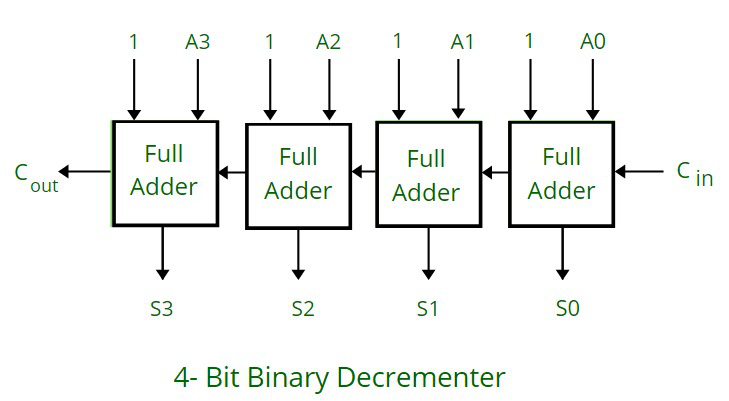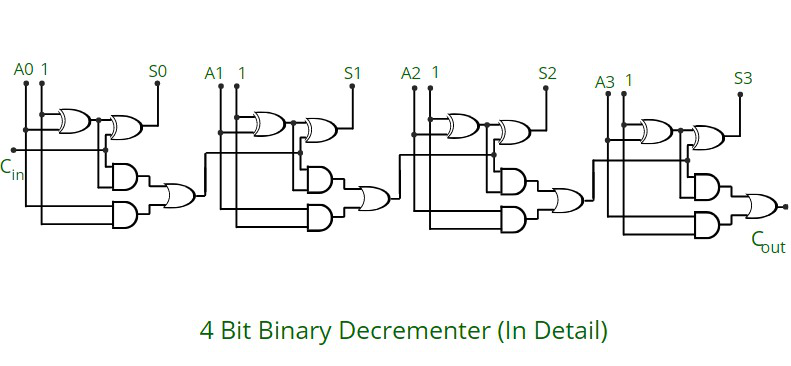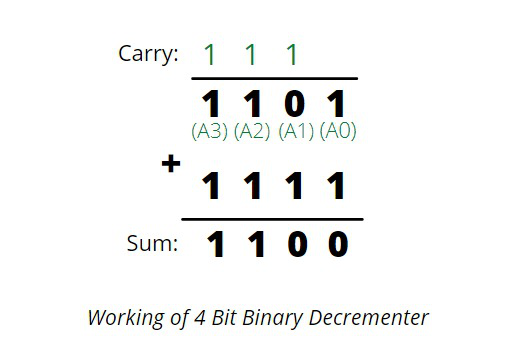Open in App
Not now

# 4 Bit Binary Decrementer

• Difficulty Level : Easy
• Last Updated : 05 May, 2021

What is 4 Bit Binary Decrementer ?
It subtracts 1 binary value from the existing binary value stored in the register or in other words we can simply say that it decreases the existing value stored in the register by 1.
For any n- bit binary decrementer, ‘n’ refers to the storage capacity of the register which needs to be decremented by 1. So we require ‘n’ number of full adders. Thus, in case of 4 bit binary decrementer we require 4 full adders.Working:

• It consists of 4 full adders, connected one after the other. Each full adder has 3 inputs (carry input, 1, A) and 2 outputs (carry output and S)
• A full adder basic consist of 2 half adders and an OR gate.
• The carry(C) from previous full adder is propagated to the next full adder. So carry output from one full adder becomes one of the three input of the next full adder.
• It follows the concept of 2’s complement, so we take 1 as input in all 4 full adder as seen from the above diagram.
• So we add 1111 in order to subtract 1.• This is because our main motive is to subtract 1 which in 4 bit representation is 0001
• Representing it in 1’s complement will give: 1110
• Representing it in 2’s complement (adding 1 to 1’s complement) will give: 1111
• This is the reason why input 1111 is given to get a decremented output in 4 bit binary decrementer.

```    In 4 bit representation           In 1's complement           In 2's complement
1 -------------------------> 0001 ----------------------> 1110 ---------------------> 1111
```Example:

```(Refer to the circuit diagram from right to left for better understanding)

1. Input: 1010  ----> After using 4 bit binary decrementer ----> Output: 1001

1 0 1 0       (Comparing from the circuit 1 0 1 0 is A3, A2, A1, A0 respectively)
+ 1 1 1 1       (1 1 1 1 is added as seen in the diagram also, in each full adder 1 is taken as input)
________
1 0 1 1       ( 1 0 1 1 , in the diagram are S3, S2, S1, S0 respectively)
________
```
```2. Input: 0010  ----> After using 4 bit binary decrementer ----> Output: 0001

1 0 1 0
+ 1 1 1 1
________
0 0 0 1
________
```
```3. Input: 0011  ----> After using 4 bit binary decrementer ----> Output: 0001

0 0 1 1
+ 1 1 1 1
_________
0 0 1 0
_________
```
My Personal Notes arrow_drop_up
Related Articles